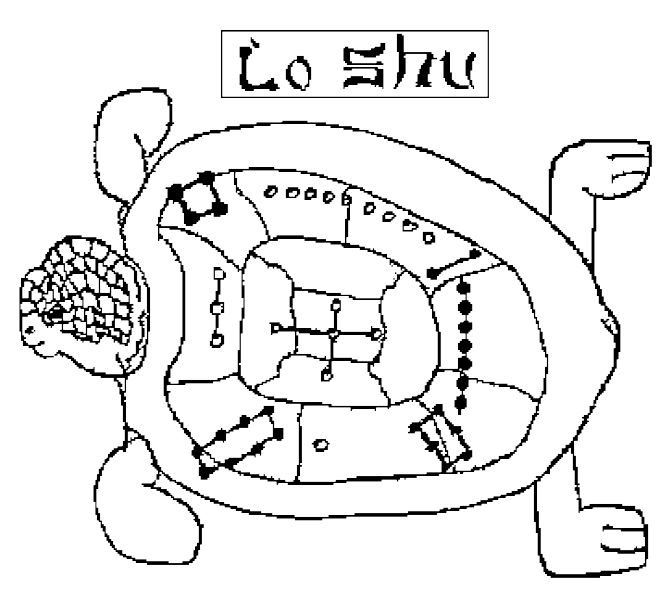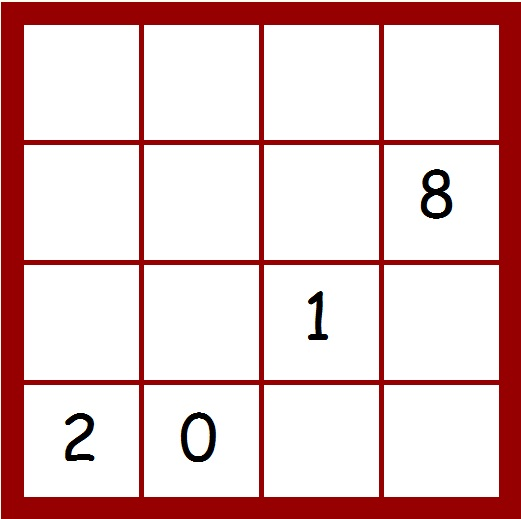Quick Links# Monthly Maths Puzzle - February 2018

###Friday February 16th is Chinese New Year – the year of the Dog.

The famous Lo-Shu magic square from ancient China is said to be the first example of a ‘magic’ square. In a magic square, the figures in each vertical, horizontal, and diagonal row add up to the same value.

### This month’s challenge is to complete the magic squareThere will be a different number from 0 to 15 in each square. Four numbers have already been placed.

The remaining twelve numbers must be arranged so that a magic square is formed; the sum of the numbers in each row, each column, and each long diagonal should be equal to 30.

••••